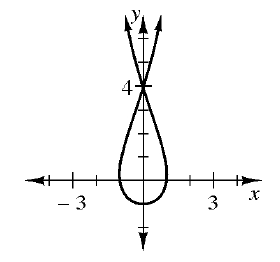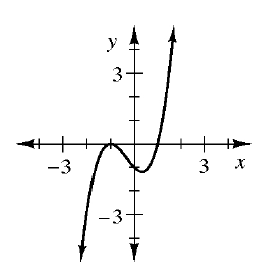### Home > CC4 > Chapter 1 > Lesson 1.2.2 > Problem1-48

1-48.

Find the $x$ and $y$intercepts for the graphs of the relationships in problem 1-47. Homework Help ✎

See the graphs below from the previous problem. Read the $x$ and $y$ intercepts off the graphs and tables.

1.1.  input $\boldsymbol {(x)}$ output $\boldsymbol {(y)}$ $-3$ $19$ $5$ $19$ $19$ $0$ $0$ $-3$
1.  input $\boldsymbol {(x)}$ $7$ $–2$ $0$ $7$ $4$ output $\boldsymbol {(y)}$ $10$ $0$ $10$ $3$ $0$
1.